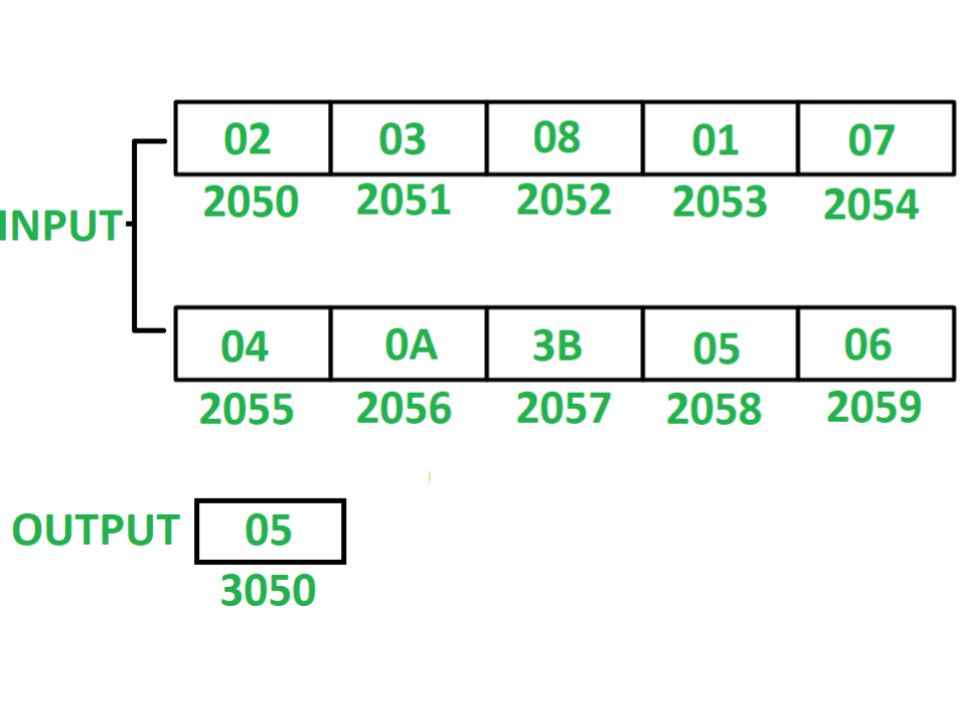GFG App
Open AppBrowser
Continue

# 8085 program to count total even numbers in series of 10 numbers

Program – Write an assembly language program in 8085 microprocessor to count even numbers in a series of 10 numbers.

Example –Assumption – Ten 8-bit numbers are stored from starting memory location 2050. Value of count is stored at memory location 3050.

Algorithm –

1. Initialize register H with 20 and register L with 4F so that indirect memory points to memory location 204F.
2. Initialize register C with 00 and register D with 0A.
3. Increment indirect memory by 1.
4. Store value of M in accumulator A.
5. Check whether the content in A is even or odd by performing AND operation of A with 01.
6. If content of A is 00 after AND operation then the number scanned was even, If so then increment C by 01 else if content of A is 01 after AND operation then number scanned was odd, If so then decrements D by 01.
7. Check if zero flag is not set i.e. ZF = 0 then jump to step 3 otherwise store value of C at memory location 3050.

Program (Using ANI Instruction) –

Explanation – Registers A, B, C, D, H, L are used for general purpose.

1. LXI H 204F: assign 20 to H and 4F to L.
2. MVI C, 00: assign 00 to C.
3. MVI D, 0A: assign 0A to D.
4. INX H: increment indirect memory location M by 01.
5. MOV A, M: move content of M to A.
6. ANI 01: perform AND operation of A with 01 and store the result in A.
7. JNZ 200F: jump if ZF = 0 to memory location 200F.
8. INR C: increment C by 01.
9. DCR D: decrements D by 01.
10. JNZ 2007: jump if ZF = 0 to memory location 2007.
11. MOV A, C: moves the content of C to A.
12. STA 3050: store the content of A to memory location 3050.
13. HLT: stops executing the program and halts any further execution.

### Alternative Approach (Using Rotate Instructions):

For a given set of numbers, If the Least Significant Bit(LSB) of each number’s Binary representation is 1, then the given number is assured to be an Odd Number.

But if the Least Significant Bit (LSB) of each number’s binary representation is 0, then the given number is assured to be an Even Number.

Hence, here we will use Rotate Instructions of 8085 Microprocessor. i.e. RAR Instruction (Rotate Accumulator Right involving Carry flag in Rotation).

If we rotate the given binary number in the right direction by 1-bit then we will get the carry a flag as Zero or One.

If we get Carry Flag = 1, then the given number is ODD, Otherwise EVEN.

My Personal Notes arrow_drop_up